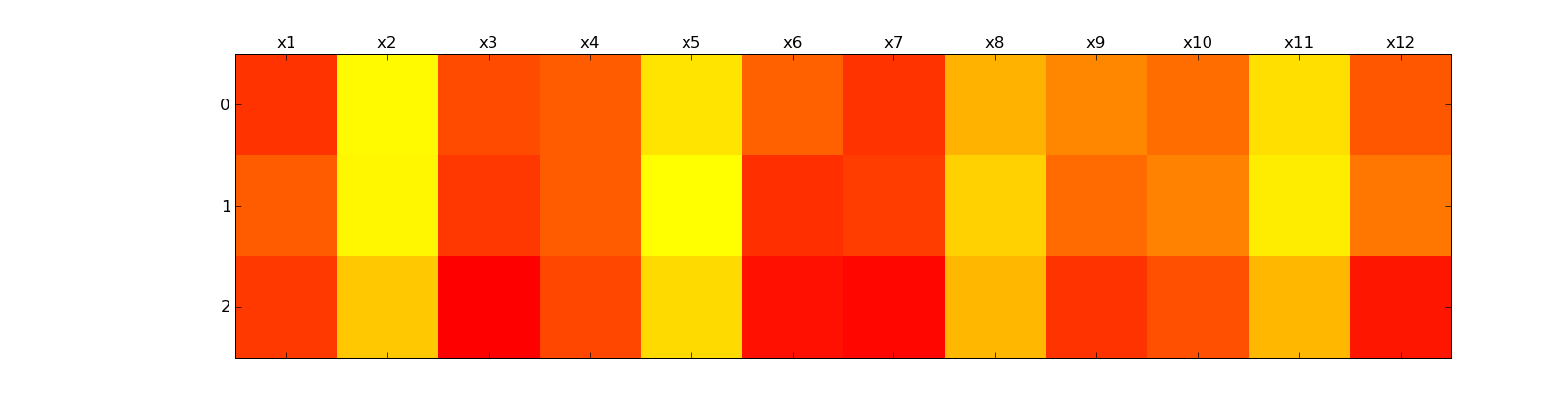# matshow

Friends,
I have a matrix data and i used matshow() function to plot. The plot is attached.

1. After plotting the data, i used xticks() function to change the x-axis tick labels from x1 to x12 ( figure attached). Similarly I want to change the y-axis tick labels into as A,B and C, instead of 0,1,2 (in the attached figure) but when i use yticks() function as below, it dosent happen, instead it changes the plot.
yticks( arange(3), (‘A’,‘B’,‘C’))
2. I used the colormap cm.autumn to create the plot. Suppose if i want to generate the same plot with green,black, red combination, how should i specify the colors in matshow.

BalaFriends,

I have a matrix data and i used matshow() function to plot. The plot is
attached.

1) After plotting the data, i used xticks() function to change the x-axis
tick labels from x1 to x12 ( figure attached). Similarly I want to change
the y-axis tick labels into as A,B and C, instead of 0,1,2 (in the attached
figure) but when i use yticks() function as below, it dosent happen, instead
it changes the plot.

yticks( arange(3), ('A','B','C'))

Can you post a complete code (a simplified version that reproduces the
problem)? I have done some simple tests but it worked as expected.

2) I used the colormap cm.autumn to create the plot. Suppose if i want to
generate the same plot with green,black, red combination, how should i
specify the colors in matshow.

If none of the mpl's colormaps fits your need, you need to create your
own colormap.

http://www.scipy.org/Cookbook/Matplotlib/Show_colormaps

-JJ

···

On Thu, Sep 17, 2009 at 2:49 AM, Bala subramanian <bala.biophysics@...287...> wrote: